Search IntMath
Close

# IntMath Newsletter: Derivative graphs, roller door problem, online math in remote India

By Murray Bourne, 05 Dec 2012

5 Dec 2012

1. Derivative graphs interactive
2. Roller door problem
3. Online math education for Ajab, Gujurat
4. Math puzzles
5. Friday math movies
6. Final thought - candles

## 1. Derivative graphs interactive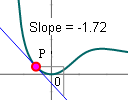Calculus can be a bit of a mystery at first. Explore these graphs to get a better idea of what differentiation actually means. Derivative graphs interactive

## 2. Roller Door problem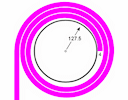Here's a real-life application of calculus posed by a roller door engineer. I actually made an error when first writing the article. I should have done an estimation first, something I always preach. (My graph worked fine, so I thought all was good. I'd used the correct formula for the graph, but not for the integration.)

## 3. Online math education for Ajab, GujaratStudents in Ajab village, India, enjoy a math lesson via Web conference from the US. Online math education for Ajab, Gujarat

## 4. Math puzzles

(a) Last puzzle: The puzzle in the last Newsletter asked about the number of messages you can send using a flashlight.

The correct answer was 30 messages. People who got it correct and showed working were: Soutrik, Pradyut De, Guido, Nicos Mavrommatis, Thomas A Buckley and Rosa.

(b) New puzzle: What is the value of the following?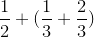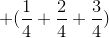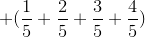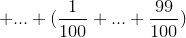You can respond in the comments.

## 5. Friday math movies(a) How things in nature tend to sync up Well-known mathematician Steven Strogatz talks about how flocking behavior naturally occurs - and some mathematical reasons for it. Friday math movie: How things in nature tend to sync up(b) Science is for everyone, kids included Meet 12-year-old Amy O'Toole presenting a scientific paper that starts, "Once upon a time..." Friday math movie: Science is for everyone, kids included

## 6. Final thought - candles

This quote suggests it's up to us to make sure we know what's going on.

If you're not lighting any candles, don't complain about being in the dark. [Anon]

Until next time, enjoy whatever you learn.

### Comment Preview

HTML: You can use simple tags like <b>, <a href="...">, etc.

To enter math, you can can either:

1. Use simple calculator-like input in the following format (surround your math in backticks, or qq on tablet or phone):
a^2 = sqrt(b^2 + c^2)
(See more on ASCIIMath syntax); or
2. Use simple LaTeX in the following format. Surround your math with $$ and $$.
$$\int g dx = \sqrt{\frac{a}{b}}$$
(This is standard simple LaTeX.)

NOTE: You can mix both types of math entry in your comment.